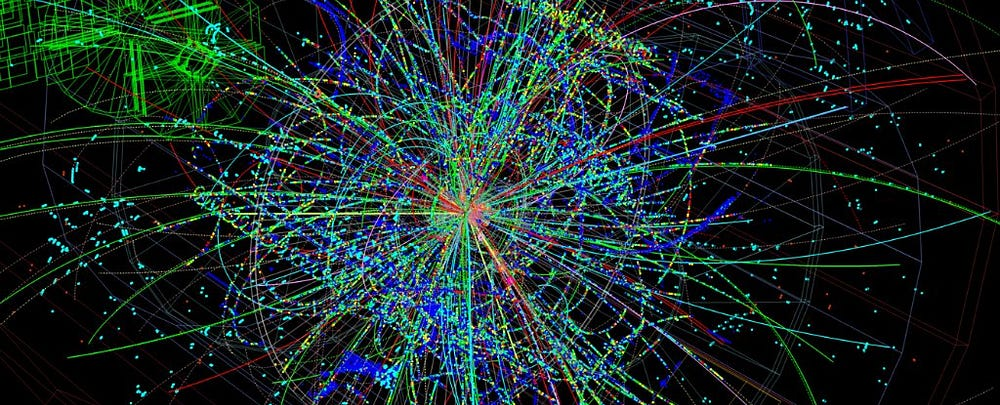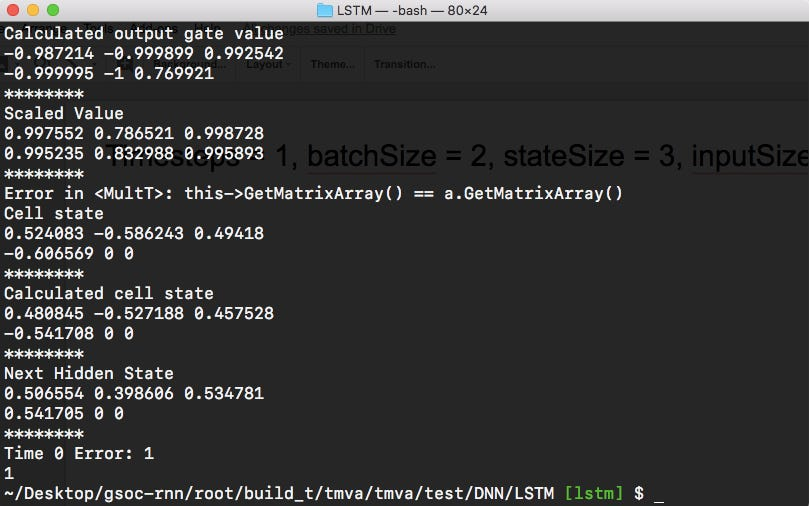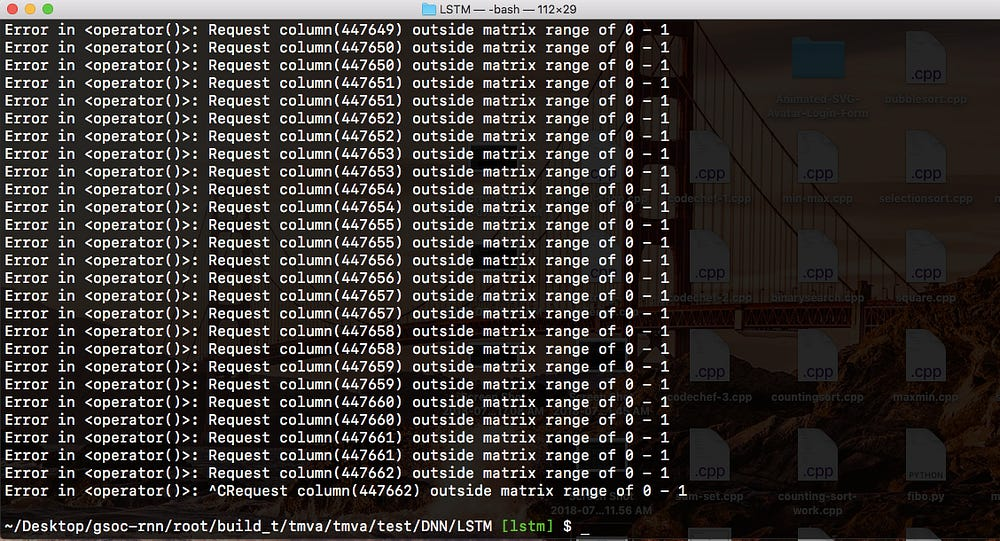# GSoC 2018: Performance of LSTM Network — Part V

## Performance of LSTM features: Forward propagation and backward propagation.

In this blogpost, I’ll be sharing results obtained during testing session of forward propagation and backward propagation. This blogpost will be a short one since, it focuses more on result.

### Forward Propagation Test Results:

For TestLSTMForwardPass.cxx : timeSteps = 1, batchSize = 2, stateSize = 3, inputSize = 2. Inputs and weights (input and state) are initialised randomly.

For TestLSTMForwardPassCpu.cxx : timeSteps = 1, batchSize = 2, stateSize = 3, inputSize = 2. Inputs and weights (input and state) are initialised randomly.Getting error as 1.0 during forward pass LSTM-CPU. Running second or third time gives error ~ 0.90

### Backpropagation Test Results:

For TestLSTMBackpropagation.cxx : timeSteps = 1, batchSize = 2, stateSize = 1, inputSize= 10, learningRate = 1e-5.The current implementation when tested, goes into infinite loop. It is difficult to debug where the problem is occurring.

For TestLSTMBackpropagationCpu.cxx : timeSteps = 1, batchSize = 2, stateSize = 1, inputSize= 10, learningRate = 1e-5 and using random inputs.